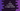# C program to check if a number is magic number or not### C program to find if a number is magic number or not :

This tutorial is to check if a number is magic number or not in c programming language. A magic number is a number which is equal to the product of sum of all digits of a number and reverse of this sum. For example, 1729 is a magic number. The sum of all digits of the number is 19. Reverse of this number 91. Product of these values is 19 * 91 = 1729. So, this is a magic number. Let’s take a look into the program first :

### C program :

``````#include

//6
int findReverse(int n) {
int reverseNumber = 0;

while (n > 0) {
reverseNumber = (reverseNumber * 10) + (n % 10);
n = n / 10;
}

return reverseNumber;
}

//4
int findSum(int n){
int sum = 0;

while (n > 0) {
sum = sum + (n % 10);
n = n / 10;
}

return sum;
}

int main(){
//1
int number;
int sum,reverseSum;

//2
printf("Enter the number : ");
scanf("%d",&number);

//3
sum = findSum(number);

//5
reverseSum = findReverse(sum);

//7
if(sum * reverseSum == number){
printf("%d is a magic number.",number);
}else{
printf("%d is not a magic number.",number);
}

}``````

### Explanation :

_The commented numbers in the above program is same as the Step number below _

1. First create integer variables to store the number, sum of the number and reverse of the sum of the number.
2. Ask the user to enter the number and store it in number variable.
3. Find the sum of each digits of the number and store it in sum variable.
4. The function findSum(int n) is used to find the sum of all the digits. It takes one integer as input and returns the sum of the digits.
5. Now create the reverse of the sum we have found on last step. Store the value in reverseSum variable.
6. The function findReverse(int n) is used to find the reverse. It also takes one integer as input and returns the reverse of that number.
7. Now, check if the product of sum and reverseSum is equal to number or not. If yes, then it is a magic number.Print out the result.

### Sample Outputs :

``````Enter the number : 1223
1223 is not a magic number

Enter the number : 1729
1729 is a magic number``````

### Where is the color and why codevscolor ?

Long story short, I love paintings and I paint on weekends. We(me and my wife) have one Youtube channel. Don't forget to subscribe ❤️❤️🤩